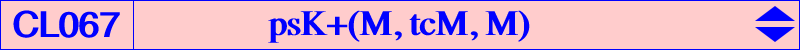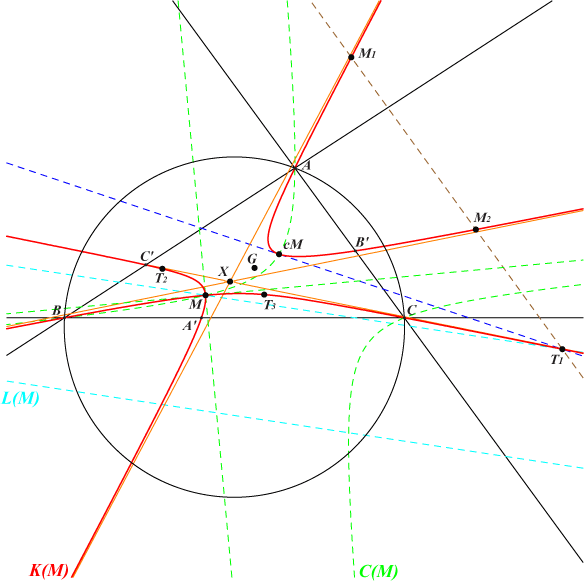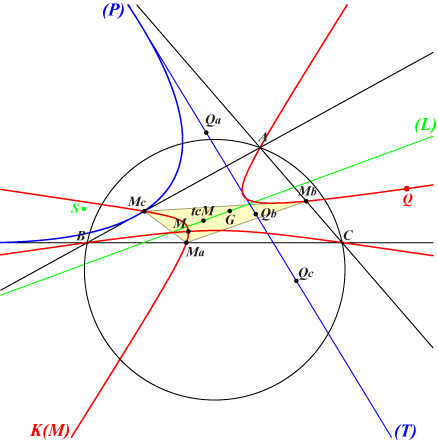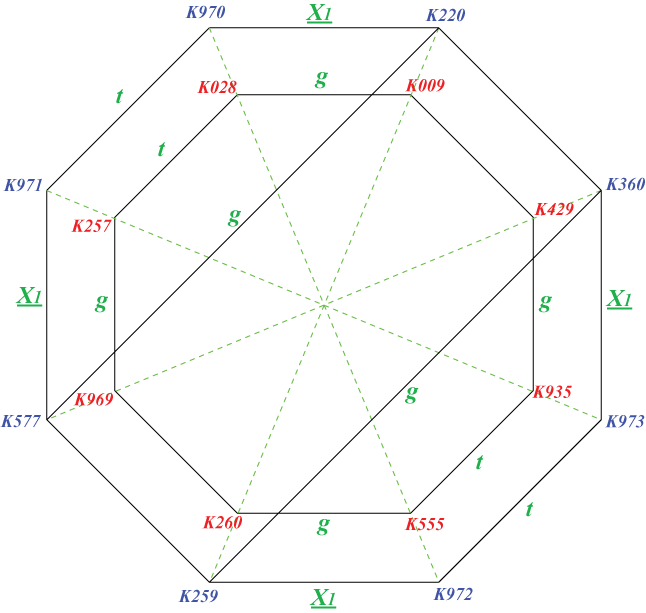Let M be a point and denote by K(M) the nodal cubic psK+(M, tcM, M). With M = u : v : w, the equation of K(M) is : ∑ u (v + w)[(u + w) y – (u + v) z] y z = (u – v) (v – w) (w – u) x y z When M = G, K(M) is the union of the medians of ABC. When M lies on a sideline or on a median of ABC, K(M) splits into this same line and a (possibly decomposed) conic. This is excluded in the sequel. *** Geometric properties • The node of K(M) is M with nodal tangents parallel to the asymptotes of the circum-conic C(M) passing through M and cM. These nodal tangents are perpendicular if and only if M lies on the Lucas cubic K007. • K(M) has three asymptotes concurring at the midpoint X of GM. These are parallel to those of pK(M x ccM, M), pK(ctM, cM), pK(cM x ccM, aM) and more generally to those of a pK with pole on psK(ctM x ccM, G, atM) and pivot on the complement of psK(ctM x ccM, G, M). • K(M) is globally invariant under the product of M-isoconjugation and tcM-Ceva conjugation, in both orders. • K(M) passes through A, B, C, M, cM, the vertices or the cevian triangle of tcM, the two points M1, M2 deduced from the transformations above . M1 = M ÷ (tcM / cM) and M2 = tcM / (M ÷ cM) where ÷ and / denote M-isoconjugation and tcM-Ceva conjugation respectively. This can be repeated thus giving other points on K(M). The tangents at A, B, C to K(M) concur at the pseudo-isopivot which is ctM = M ÷ tcM. • The tangential T1 of cM lies on the line M1M2 and on the parallel at M to the trilinear polar L(M) of M. It folllows that T2 = M ÷ (tcM / T1) and T3 = tcM / (M ÷ T1) are two other points on K(M). Note that cM, M1, T3 and cM, M2, T2 are two triads of collinear points on K(M). • If P = p : q : r is any point different of M then Q = (v+w) (q u-r u-p v-r v+p w+q w) / (r v-q w) : : lies on K(M). Q = cM x aZ ÷ Z where Z is the isotomic conjugate of the trilinear pole of the line MP.Construction of K(M)Let M be a point different of G. Denote by MaMbMc the cevian triangle of tcM and by (L) the line passing through G and tcM. The trilinear pole S of (L) lies on the Steiner ellipse hence the inscribed conic with perspector S is a parabola (P). A variable tangent (T) to (P) meets the lines MMa, MMb, MMc at Qa, Qb, Qc respectively. The triangles ABC and QaQbQc are perspective at Q which lies on K(M). Note that the nodal tangents at M to K(M) are those drawn from M to (P). *** When M = X(1), K(M) is the cubic K974 = psK(X1, X86, X1) and (P) is the Kiepert parabola. More generally, for any M on the Wallace hyperbola, (P) is the Kiepert parabola again and tcM lies on the line X(2)X(6).Recall that the Wallace hyperbola is the diagonal rectangular hyperbola passing through the in/excenters of ABC, G and many other centers such as X(i) for i = 20, 63, 147, 194, 487, 488, 616, 617, 627, 628, 1670, 1671, 1764, 2128, 2582, 2583, 2896, etc. Another example is K(X20) = K041.Related cubics • Under isotomic conjugation, K(M) is transformed into a cubic of the same type namely K(tM) = psK+(tM, tctM, tM). • Under M-isoconjugation and/or tcM-Ceva conjugation, K(M) is transformed into another nodal cubic K'(M) = psK(M, tcM, G). • The barycentric quotient of K(M) by M is psK(tM, tcM x tM, G). *** Strong and weak cubics When M is a strong (resp. weak) center, K(M) is a strong (resp. weak) cubic. Under the symbolic substitution SS{a -> a^2}, a weak cubic K(M) is transformed into a strong cubic K(M). For example, the weak cubic psK+(X8, X75, X1) is associated with the strong cubic psK+(X69, X76, X6) = K257. *** A selection of cubics The following table presents a selection of cubics K(M) according to the node M. A weak cubic (highlighted in green) is associated with a strong cubic (highlighted in pink). Two strong cubics (in yellow) can also be associated.M K(M) X Xi on K(M) for i = associated K(M) X1 K974 = psK(X1, X86, X1) X551 1, 10, 21, 274 K975 X3 K976 = psK(X3, X95, X3) X549 3, 5, 54, 276 psK(X9, X32008, X9) X4 K028 = psK(X4, X264, X3) X381 3, 4, 8, 76, 847, etc K970 X6 K975 = psK(X6, X83, X6) X5480 6, 141, 308, 1176 K974 X7 K970 = psK(X7, X85, X7) X6173 7, 9, 75, 77, 342 K028 X8 K971 = psK(X8, X75, X1) X3679 1, 8, 85, 271, 318 K257 X20 K041 = psK(X20, X69, X4) X376 4, 20, 279, 280, 9292 psK(X144, X8, X7) X66 psK(X66, X40421, X66) ? 66, 69, 160, 206, 1502 X69 K257 = psK(X69, X76, X6) X599 6, 7, 69, 264, 9723, 15394 K971 X75 psK(X75, X274, X37) X4688 37, 75, 86, 1441, 2322 psK(X76, X308, X39) X76 psK(X76, X308, X39) X9466 39, 76, 83, 1235 psK(X75, X274, X37) X100 psK(X100, X4998, X11) X6174 11, 100, 108, 658 psK(X110, X18020, X107) X189 psK(X189, X34404, X63) ? 63, 189, 223, 312 psK(X13575, ?, X22) X194 psK(X194, X6, X76) X7757 76, 194, 330, 3224 psK(X192, X1, X75) X253 psK(X253, X34403, X69) ? 69, 253, 346, 347, 1249 psK(X10405, ?, X8) X264 psK(X264, X276, X95) ? 95, 216, 264, 311 psK(X85, X31618, X85)Remarks : • two isotomic conjugates M and tM correspond to K(M) and K(tM) = t K(M). See K028 and K257 for example with M = X4 and X69. • the "orange" points M lie on K007 hence the nodal tangents at M to K(M) are perpendicular. The pseudo-pivot tcM lies on K184. *** The diagram below shows several cubics K(M) and other related cubics obtained through usual transformations. A blue cubic is weak and the red nearby cubic is that obtained under SS{a -> a^2}. Beware not all the cubics are members of the class. Two cubics of the same color in a diagonal of an octogon have the same pseudo-pole Ω and the same pseudo-pivot P. They are exchanged by Ω-isoconjugation and by P-Ceva conjugation. For example, K009 = psK(X184, X2, X3) and K260 = psK(X184, X2, X6). As usual, g and t denote isogonal and isotomic conjugations. X1 denotes X1-isoconjugation i.e. isoconjugation with pole the incenter X1.Remarks : • two cubics of the same color can be related either by other isoconjugations or by some barycentric products. For example, K260 is the X3-isoconjugate of K257, the X32-isoconjugate of K429 and the barycentric products X(3) x K028, X(6) x K935. • the cubics K620, K724, K967 = g K971, K1069 = t K220 can also be related similarly to one or several of the sixteen cubics in the diagram above. • the following table gives the unlisted isogonal and isotomic transforms of the cubics of the diagram.cubic type X(i) on the cubic g K970 psK(X2175, X1, X31) 31, 33, 55, 57, 2188, 3158, 6654, 40172, 42622 g K972 psK(X2175, X1, X6) 6, 9, 48, 1253, 1419, 2191, 2331, 27958, 35192, 38266 g K973 psK(X1397, X57, X6) 6, 19, 269, 604, 1743, 21059 t K009 psK(X18022, X18022, X69) 69, 264, 1502, 3596, 17233, 17907, 34208, 40810, 52551, 55553 t K259 psK(X6063, X6063, X7) 7, 75, 264, 344 t K260 psK(X18022, X18022, X4) 4, 76, 331, 7763, 18027, 34403, 40421, 40804, 51257, 56666 t K360 psK(X3596, X76, X69) 69, 75, 346, 3596, 4373, 50560 t K429 psK(X40050, X1502, X76) 76, 315, 2996, 3926, 40050 t K577 psK(X3596, X76, X2) 2, 312, 6063, 7017, 44189, 44723, 52651 t K969 psK(X40050, X1502, X2) 2, 305, 6063, 18022